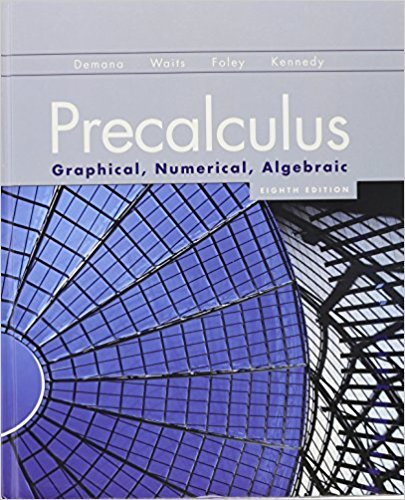×
×

# Solutions for Chapter 5: Analytic Trigonometry## Full solutions for Precalculus: Graphical, Numerical, Algebraic | 8th Edition

ISBN: 9780321656933Solutions for Chapter 5: Analytic Trigonometry

Solutions for Chapter 5
4 5 0 345 Reviews
17
0
##### ISBN: 9780321656933

Precalculus: Graphical, Numerical, Algebraic was written by and is associated to the ISBN: 9780321656933. Chapter 5: Analytic Trigonometry includes 83 full step-by-step solutions. This textbook survival guide was created for the textbook: Precalculus: Graphical, Numerical, Algebraic, edition: 8th Edition. This expansive textbook survival guide covers the following chapters and their solutions. Since 83 problems in chapter 5: Analytic Trigonometry have been answered, more than 45502 students have viewed full step-by-step solutions from this chapter.

Key Calculus Terms and definitions covered in this textbook
• Acute triangle

A triangle in which all angles measure less than 90°

• Augmented matrix

A matrix that represents a system of equations.

• Damping factor

The factor Ae-a in an equation such as y = Ae-at cos bt

• Domain of a function

The set of all input values for a function

• Fundamental

Theorem of Algebra A polynomial function of degree has n complex zeros (counting multiplicity).

• Midpoint (in Cartesian space)

For the line segment with endpoints (x 1, y1, z 1) and (x2, y2, z2), ax 1 + x 22 ,y1 + y22 ,z 1 + z 22 b

• Minute

Angle measure equal to 1/60 of a degree.

• Multiplicative inverse of a complex number

The reciprocal of a + bi, or 1 a + bi = a a2 + b2- ba2 + b2 i

• Multiplicative inverse of a matrix

See Inverse of a matrix

• Order of magnitude (of n)

log n.

• Real number

Any number that can be written as a decimal.

• Second quartile

See Quartile.

• Semimajor axis

The distance from the center to a vertex of an ellipse.

• Symmetric matrix

A matrix A = [aij] with the property aij = aji for all i and j

• Trigonometric form of a complex number

r(cos ? + i sin ?)

• Union of two sets A and B

The set of all elements that belong to A or B or both.

• Viewing window

The rectangular portion of the coordinate plane specified by the dimensions [Xmin, Xmax] by [Ymin, Ymax].

• Weights

See Weighted mean.

• xz-plane

The points x, 0, z in Cartesian space.

• Zero factorial

See n factorial.

×